Home > Tutorials > Excel Markbook

# Excel Markbook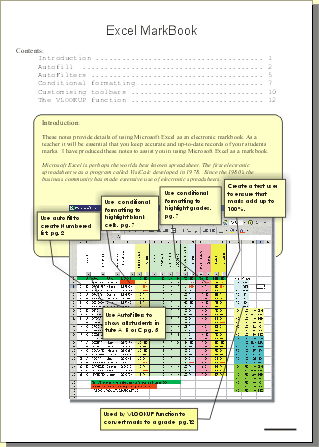Since I wrote these notes I have learnt a few new things about using the Vlookup formula

1) You can place the vlookup table on a separate worksheet and reference it from within the formula using the name of the worksheet

2) If you place the vlookup table on a separate worksheet you don't have to state the upper left and lower right cell numbers in the formula

The result of this becomes a formula that looks like the following:

=VLOOKUP(G2,Table!\$A:\$B,2,TRUE)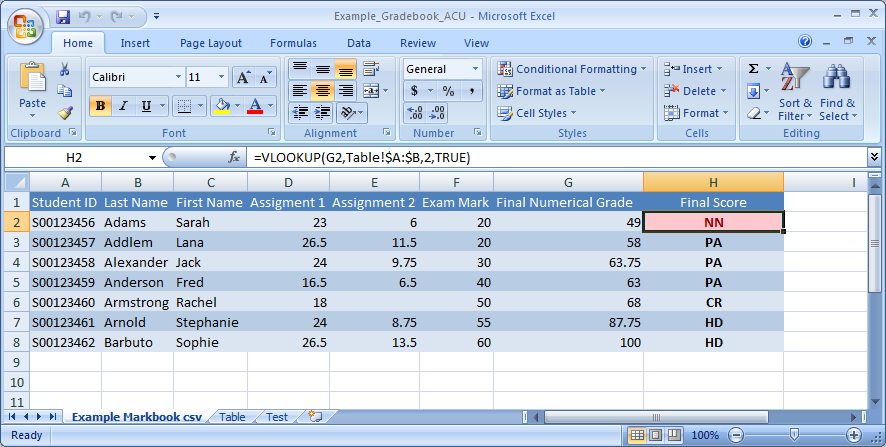Hello Rupert,

I saw your website about an Excel markbook, I'm starting to make my own and I have a query you might be able to help with.

I have attached the sheet.

I want to be able to enter "Abs" when students miss an assignment legitimately and have it not affect their term average or grade.
i.e. only average the five assignments that Fred has completed and ignore the missed one.

Do you have any idea how I can achieve this?

=ISNUMBER(A1) will return TRUE of FALSE

=IF(ISNUMBER(H5),(H5/\$H\$4)*100,0)+IF(ISNUMBER(I5),(I5/\$I\$4)*100,0) The trick is to use a sequence of if commands IF(ISNUMBER())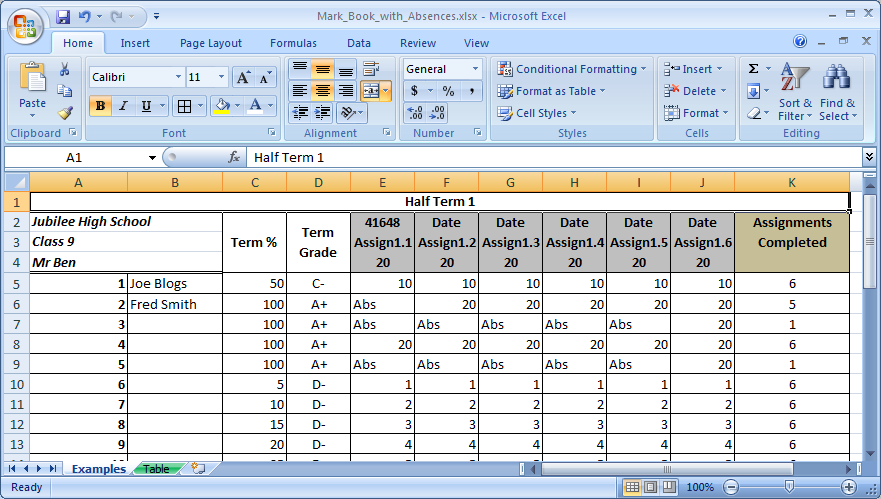Markbook Excel13 KB created 19 June 2014

Markbook ACU gradings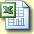14 KB xlsx file
updated 25 November 2014 thanks to Amber Van Dreven for poiniting out an issue with rounding of grades

Note: you can also use Vlookup to merge data from 2 worksheets in Excel

https://echo360.org.au/media/ac5acbc4-ca1a-4139-bf47-ecc67a508814/public

Transcript:

One of the really useful things about the VLOOKUP formula is that you can use it to combine or merge data from two worksheets.

in this example, I have a list of students and I'm using the VLOOKUP to bring data from two separate worksheets into a single combined version.

So in this worksheet I have students who are enrolled in the unit HLSC111.
In this one, I have a list of students enrolled in in NRSG222 and I want to combine them.

I need to look them up and find out the combined grades for each student.
So there's a few things to note The first thing is that it's very important that the Key Field which is the student number, is in column A in the sheet that you're looking into or looking up. So you'll notice that the student numbers are in column A in this sheet and also in NRSG222.

The Key Field does not have to be in column A in the combined sheet. So in this example, I've got first name and last name in the first two columns. And the Key Field is in column C. The formula sits here and this is what it looks like =VLOOKUP(C2,HLSC111!A:B,2,FALSE).

What it's saying is look up the Key Field in cell C2, look in the worksheet HLSC111 and notice there is an exclamation mark [after the sheet name]. So this is the name of this worksheet. Look in both columns in HLSC111. So I'm using column A, and column B and return whatever's in the second column.

Now the word FALSE means [use] an exact match. So it means the VLOOKUP needs to find an exact match for the student number. It's not going to use a range of numbers. And if we have an #N/A what that means is that the student was not enrolled in that unit. So in this case you can see that this student here is enrolled in NRSG222 but is not enrolled in HLSC111, so it returns #N/A.

Okay hopefully that makes sense.

It's extremely useful when you're trying to combine data and yeah, I use it a lot.
Okay thank you.
And I shall stop recording.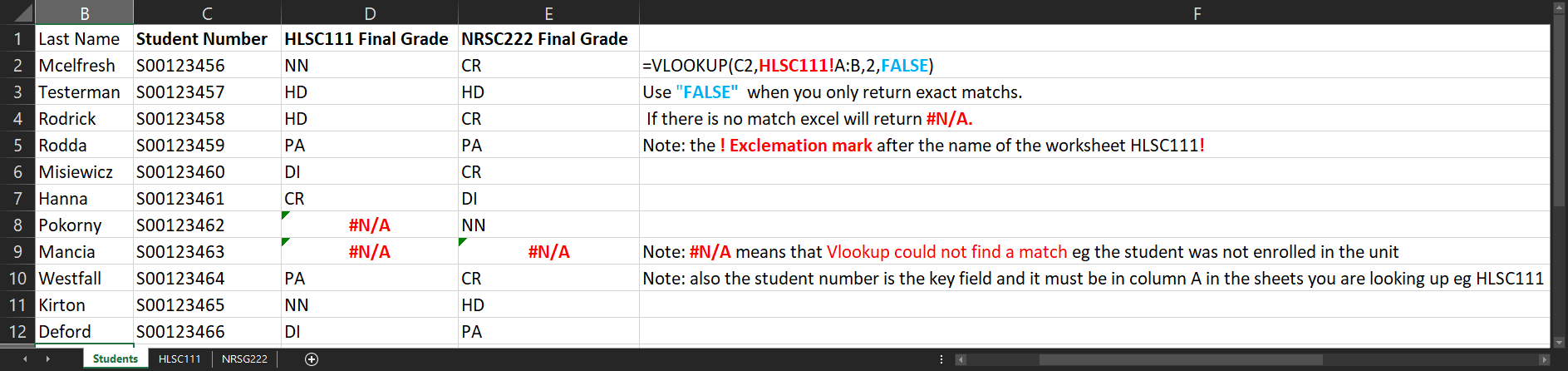APA citation:
Russell, R. (2021, September 14, 06:31 am). Excel markbook example.
Retrieved June 07, 2023, from http://www.rupert.id.au/tutorials\markbook/index.php

Last refreshed: June 07 2023. 03:27.23 pm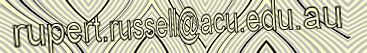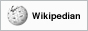This work is licensed under a Creative Commons Attribution-NonCommercial-ShareAlike 2.5 License.

950 visits since July 26, 2006